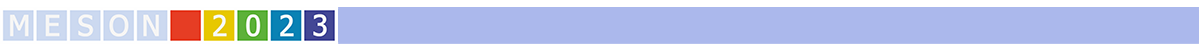#MESON2023

Jun 22 – 27, 2023
Auditorium Maximum
Europe/Warsaw timezone

## Hadron Physics results at KLOE-2

Jun 27, 2023, 12:00 PM
30m
Medium lecture hall (A and B) (Auditorium Maximum)

Plenary

### Speaker

Elena Perez del Rio (Jagiellonian University)

### Description

KLOE and KLOE-2 data are the largest dataset ever collected at an electron-positron collider operating at the $\phi$ resonance peak (almost 8 fb$^{-1}$).
The data corresponds to the production of about 24 billion of $\phi$ mesons, namely 8 billion pairs of neutral K mesons and 300 million $\eta$ mesons.
A wide hadron physics program, investigating rare meson decays, $\gamma\gamma$ interaction, and dark forces, is being carried out by the KLOE-2 Collaboration.

The $\eta$ decay into $\pi^0 \gamma \gamma$ is a test bench for various models and effective theories, like VMD (Vector Meson Dominance) or ChPT (Chiral Perturbation Theory, which predict branching ratio (BR) far from the experimental value. KLOE-2, with its highly pure $\eta$ sample produced
in $\phi \to \eta \gamma$ process, performed a new precise measurement of this BR.

KLOE-2 is currently probing a complementary model to the U boson or "dark photon", where the dark force mediator is a hypothetical leptophobic B boson that could show up in the $\phi \to \eta B\to \eta \pi^0\gamma\,, \eta \to \gamma \gamma$ channel. The preliminary upper limit on the dark $\alpha_{\rm B}$ coupling constant will be shown.

The High Energy Tagger detectors of KLOE-2 open the possibility to investigate $\pi^0$ production from $\gamma \gamma$ scattering by
tagging final-state leptons from $e^+e^- \to \gamma^{\ast}\gamma^{\ast}e^+e^-\to \pi^0 e^+e^-$ in coincidence with the $\pi^0$ in the barrel calorimeter. The preliminary measurement of the $\gamma^{\ast}\gamma^{\ast} \to \pi^0$ counting obtained by using single tagged events will be reported.

Moreover, the search for the double suppressed $\phi\rightarrow \eta\, \pi^+ \pi^-$ and the conversion $\phi\rightarrow \eta\, \mu^+ \mu^-$ decays are being performed at KLOE-2 with both $\eta \to \gamma \gamma$ and $\eta \to 3\pi^0$. Clear signals are seen for the first time.

Finally, preliminary and promising results on the $\omega$ cross-section measurement in the $e^+ e^- \to \pi^+ \pi^- \pi^0 \gamma_{\rm ISR}$ channel using the Initial State Radiation (ISR) method will be also presented.

Collaboration KLOE-2

### Primary author

Prof. Giuseppe Mandaglio (University of Messina & INFN-Catania)